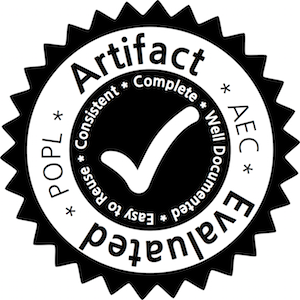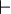Author: Damien Pous.

# Symbolic Algorithms for Language Equivalence and Kleene Algebra with Tests

This page is a web-appendix to the following paper, in Proc. POPL'15. (.pdf)## Abstract

We propose algorithms for checking language equivalence of finite automata over a large alphabet. We use symbolic automata, where the transition function is compactly represented using (multi-terminal) binary decision diagrams (BDD). The key idea consists in computing a bisimulation by exploring reachable pairs symbolically, so as to avoid redundancies. This idea can be combined with already existing optimisations, and we show in particular a nice integration with the disjoint sets forest data-structure from Hopcroft and Karp's standard algorithm. Then we consider Kleene algebra with tests (KAT), an algebraic theory that can be used for verification in various domains ranging from compiler optimisation to network programming analysis. This theory is decidable by reduction to language equivalence of automata on guarded strings, a particular kind of automata that have exponentially large alphabets. We propose several methods allowing to construct symbolic automata out of KAT expressions, based either on Brzozowski's derivatives or on standard automata constructions. All in all, this results in efficient algorithms for deciding equivalence of KAT expressions.

## Code releases (OCaml)

The library is distributed using opam, and split into two packages.

## Interactive applet (thanks to js_of_ocaml)

The various algorithms can be tested online, using the following applet. It suffices to write your favourite KAT expressions in the two text fields below. See below for syntax. Guarded strings appearing below the textboxes are typical counter-examples, when one or the other inclusion does not hold. The corresponding automata are depicted, with a trace of the execution of the algorithm represented with violet lines (for the naive algorithm) or arrows (for the Hopcroft and Karp variant). Note that when only one inclusion holds, the depicted trace is that corresponding to the proof of that inclusion (i.e., a trace of x+y=y when x<y holds).

Blue lines (or arrows) represent pairs of states whose outputs have been checked, and whose successors have been pushed to the todo list. Violet lines represent pairs of internal BDD nodes that the algorithm did visit. Dashed blue lines represent pairs of states that could be skipped thanks to the up-to-congruence technique. A red line represent a counter-example: a pair of states with distinct ouputs, that could be reached from the initial states

 Hypotheses to eliminate First expression Second expression### Examples

Click the following examples to load them.

### Syntax

• atomic tests are characters from a to j
• atomic Kleene elements are characters from k to z
• multiplication or Boolean conjunction is implicit, by juxtaposition
• addition or Boolean disjunction is +
• Kleene star is postfix *
• Boolean negation is prefix !
• zero and one are 0 and 1

### Elimination of hypotheses

You can enter KAT hypotheses to exploit in the first textbox. They are pairs of KAT expressions related either by inclusion or by equality. Use semicolon (;) to separate pairs; only some kinds of equations can be eliminated:

• x=0, for an arbitrary expression x;
• ax=xb, ax<xb, or ax>xb, for arbitrary tests a and b, and expression x;
• ap=a, or pa=a, for arbitrary test a, but atomic variable p;
• p=q, for atomic variables p and q.